# Train Reinforcement Learning Agent in Basic Grid World

This example shows how to solve a grid world environment using reinforcement learning by training Q-learning and SARSA agents. For more information on these agents, see Q-Learning Agents and SARSA Agents.

This grid world environment has the following configuration and rules:

1. The grid world is 5-by-5 and bounded by borders, with four possible actions (North = 1, South = 2, East = 3, West = 4).

2. The agent begins from cell [2,1] (second row, first column).

3. The agent receives a reward +10 if it reaches the terminal state at cell [5,5] (blue).

4. The environment contains a special jump from cell [2,4] to cell [4,4] with a reward of +5.

5. The agent is blocked by obstacles (black cells).

6. All other actions result in –1 reward.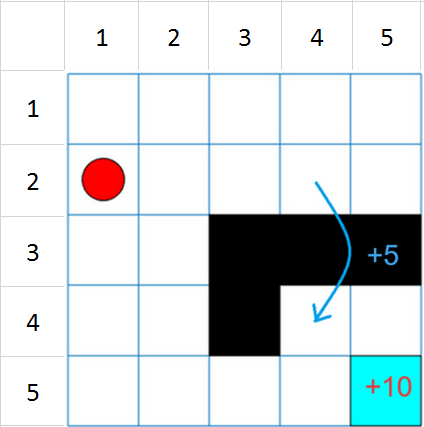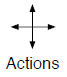### Create Grid World Environment

Create the basic grid world environment.

`env = rlPredefinedEnv("BasicGridWorld");`

To specify that the initial state of the agent is always [2,1], create a reset function that returns the state number for the initial agent state. This function is called at the start of each training episode and simulation. States are numbered starting at position [1,1]. The state number increases as you move down the first column and then down each subsequent column. Therefore, create an anonymous function handle that sets the initial state to `2`.

`env.ResetFcn = @() 2;`

Fix the random generator seed for reproducibility.

`rng(0)`

### Create Q-Learning Agent

To create a Q-learning agent, first create a Q table using the observation and action specifications from the grid world environment. Set the learning rate of the optimizer to `0.01`.

```qTable = rlTable(getObservationInfo(env), ... getActionInfo(env));```

To approximate the Q-value function within the agent, create a `rlQValueFunction` approximator object, using the table and the environment information.

```qFcnAppx = rlQValueFunction(qTable, ... getObservationInfo(env), ... getActionInfo(env));```

Next, create a Q-learning agent using the Q-value function.

`qAgent = rlQAgent(qFcnAppx);`

Configure agent options such as the epsilon-greedy exploration and the learning rate for the function approximator.

```qAgent.AgentOptions.EpsilonGreedyExploration.Epsilon = .04; qAgent.AgentOptions.CriticOptimizerOptions.LearnRate = 0.01;```

For more information on creating Q-learning agents, see `rlQAgent` and `rlQAgentOptions`.

### Train Q-Learning Agent

To train the agent, first specify the training options. For this example, use the following options:

• Train for at most 200 episodes. Specify that each episode lasts for most 50 time steps.

• Stop training when the agent receives an average cumulative reward greater than 10 over 30 consecutive episodes.

For more information, see `rlTrainingOptions`.

```trainOpts = rlTrainingOptions; trainOpts.MaxStepsPerEpisode = 50; trainOpts.MaxEpisodes= 200; trainOpts.StopTrainingCriteria = "AverageReward"; trainOpts.StopTrainingValue = 11; trainOpts.ScoreAveragingWindowLength = 30;```

Train the Q-learning agent using the `train` function. Training can take several minutes to complete. To save time while running this example, load a pretrained agent by setting `doTraining` to `false`. To train the agent yourself, set `doTraining` to `true`.

```doTraining = false; if doTraining % Train the agent. trainingStats = train(qAgent,env,trainOpts); else % Load the pretrained agent for the example. load('basicGWQAgent.mat','qAgent') end```

The Episode Manager window opens and displays the training progress.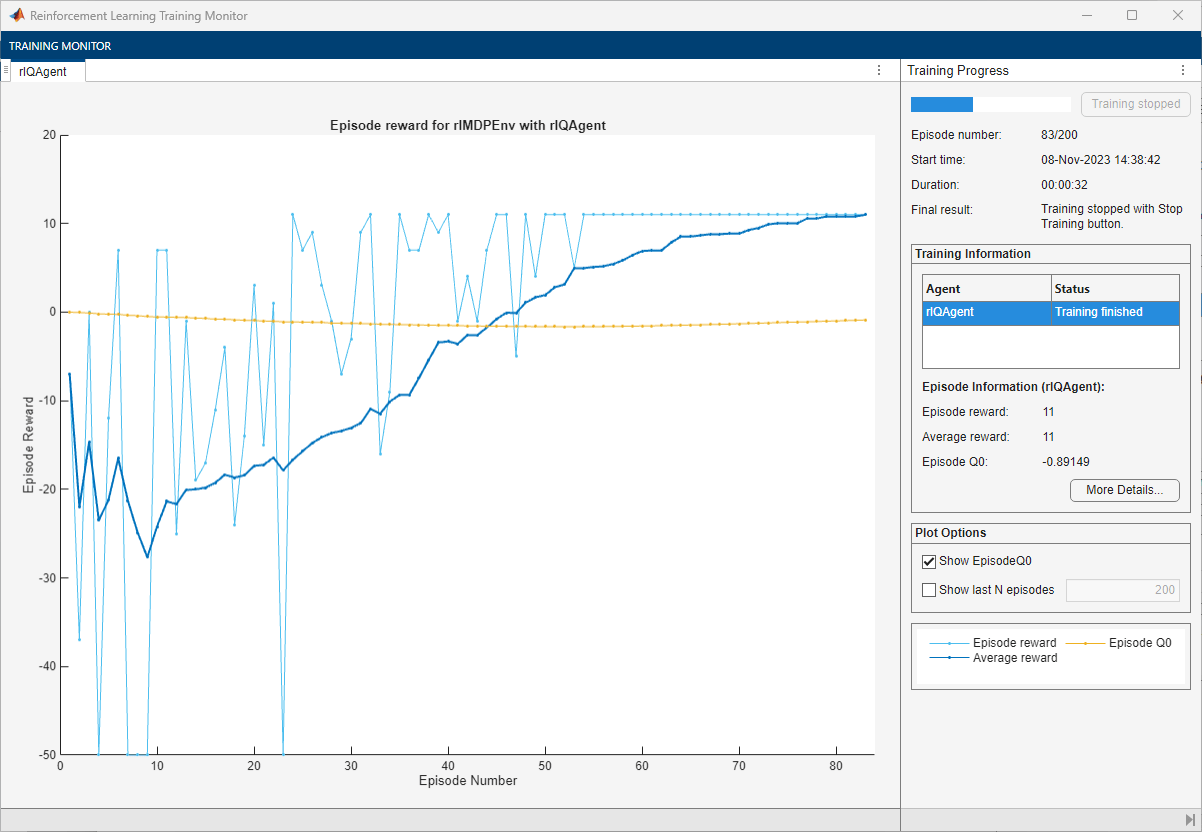### Validate Q-Learning Results

To validate the training results, simulate the agent in the training environment.

Before running the simulation, visualize the environment and configure the visualization to maintain a trace of the agent states.

```plot(env) env.Model.Viewer.ShowTrace = true; env.Model.Viewer.clearTrace;```

Simulate the agent in the environment using the `sim` function.

`sim(qAgent,env)`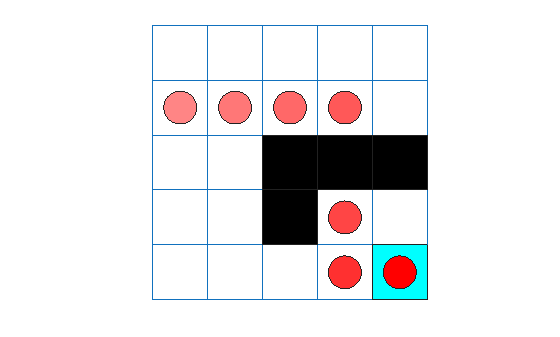The agent trace shows that the agent successfully finds the jump from cell [2,4] to cell [4,4].

### Create and Train SARSA Agent

To create a SARSA agent, use the same Q value function and epsilon-greedy configuration as for the Q-learning agent. For more information on creating SARSA agents, see `rlSARSAAgent` and `rlSARSAAgentOptions`.

```sarsaAgent = rlSARSAAgent(qFcnAppx); sarsaAgent.AgentOptions.EpsilonGreedyExploration.Epsilon = .04; sarsaAgent.AgentOptions.CriticOptimizerOptions.LearnRate = 0.01;```

Train the SARSA agent using the `train` function. Training can take several minutes to complete. To save time while running this example, load a pretrained agent by setting `doTraining` to `false`. To train the agent yourself, set `doTraining` to `true`.

```doTraining = false; if doTraining % Train the agent. trainingStats = train(sarsaAgent,env,trainOpts); else % Load the pretrained agent for the example. load('basicGWSarsaAgent.mat','sarsaAgent') end```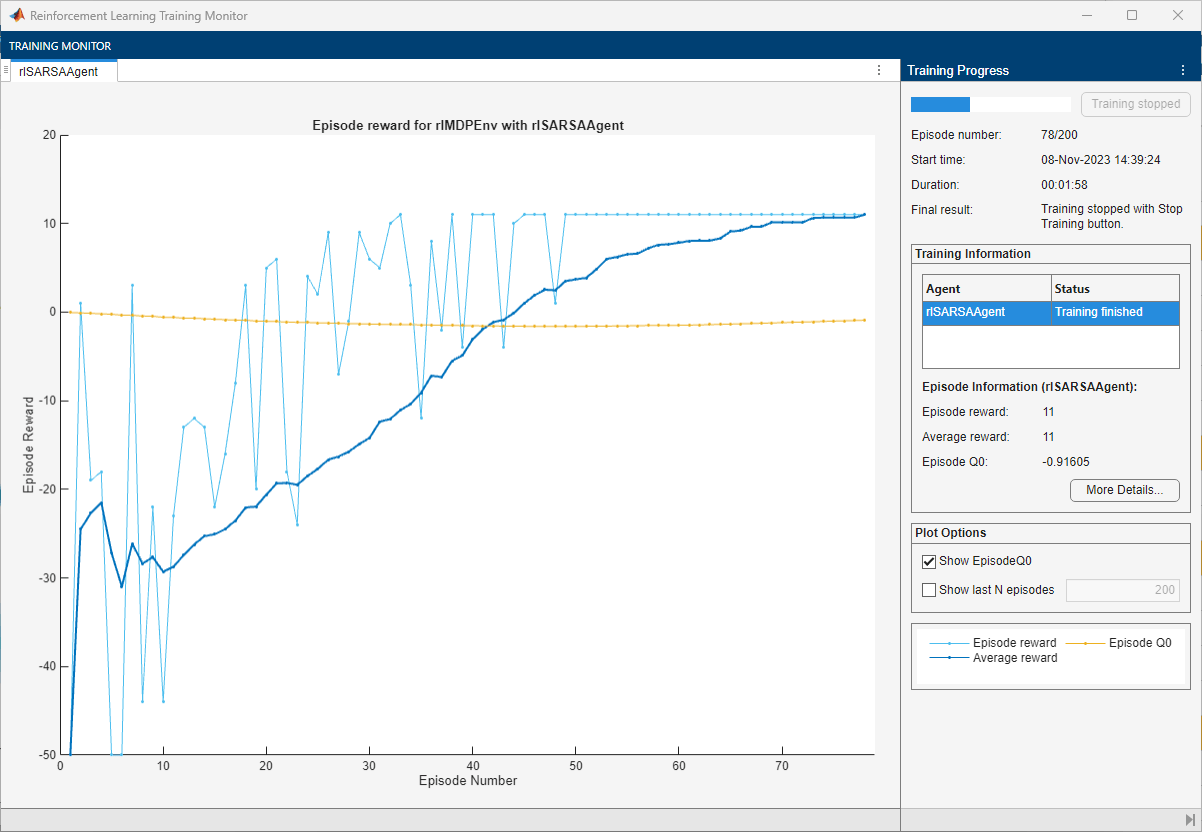### Validate SARSA Training

To validate the training results, simulate the agent in the training environment.

```plot(env) env.Model.Viewer.ShowTrace = true; env.Model.Viewer.clearTrace;```

Simulate the agent in the environment.

`sim(sarsaAgent,env)`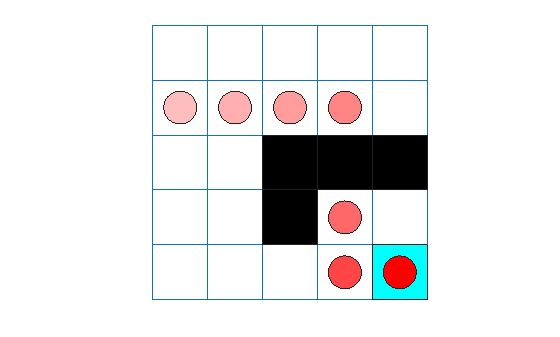The SARSA agent finds the same grid world solution as the Q-learning agent.